﻿ 结合波段选择的差分进化高光谱图像分类
«上一篇文章快速检索 高级检索

 应用科技2019, Vol. 46Issue (5): 45-50  DOI: 10.11991/yykj.2018110190

### 引用本文TIAN Hongchen, WANG Liguo, ZHAO Liang, et al. Differential evolution hyperspectral image classification combined with band selection[J]. Applied Science and Technology, 2019, 46(5): 45-50. DOI: 10.11991/yykj.201811019.### 文章历史

Differential evolution hyperspectral image classification combined with band selection
TIAN Hongchen , WANG Liguo, ZHAO Liang , CHEN Chunyu
College of Information and Communications Engineering, Harbin Engineering University, Harbin 150001, China
Abstract: Hyperspectral images have the characteristics of high dimension of data and few labeled samples, which affects the effect of existing classification methods. Aiming at this situation, we propose a semi-supervised classification algorithm combining band selection. Firstly, the band selection method is used to remove the redundant information in the hyperspectral image, reducing the complexity and improving the generalization ability. Then, the differential evolution algorithm is used to cross-mutates the unlabeled samples, and expand the labeled sample group by selecting the samples with high confidence. The experimental results show that the proposed method can effectively improve the classification accuracy and speed of the classifier under the condition of limited label samples.
Keywords: hyperspectral    hyperspectral image classification    semi-supervised classification    band selection    differential evolution    genetic algorithm    support vector machine    machine learning

1 LSTSVM算法

LSTSVM算法是在SVM的基础上找出2个相对较小且不相互平行的分类超平面，并使得每个超平面各与其中一类样本尽可能近，与另一类样本尽可能远。把2个凸优化问题转变为2个线性方程问题，降低算法复杂度，减少计算量：

 $\begin{array}{l}\left\{ \begin{array}{l} \mathop {{\rm{min}}}\limits_{{\theta _1}{r_1}} \dfrac{1}{2}{\left\| {K \left( {{{A}}, {{{C}}^{\rm{T}}}} \right){\theta _1} + {{{e}}_{{1}}}{r_1}} \right\|^2} + \dfrac{c}{2}{{\xi}} _2^T{{{\xi}} _2} \\ {\rm{s}}{\rm{.t}}{\rm{.}} - \left( {K \left( {{{B}}, {{{C}}^{\rm{T}}}} \right){\theta _1} + {{{e}}_{{2}}}{r_1}} \right) + {\xi _2} = {e_2} \\ \end{array} \right.\\ \left\{ \begin{array}{l} \mathop {{\rm{min}}}\limits_{{\theta _2}{r_2}} \dfrac{1}{2}{\left\| {K \left( {{{B}}, {{{C}}^{\rm{T}}}} \right){\theta _2} + {{{e}}_{{2}}}{r_2}} \right\|^2} + \dfrac{c}{2}{{\xi}} _1^{\rm{T}}{{{\xi}} _1} \\ {\rm{s}}{\rm{.t}}{\rm{.}} - \left( {K \left( {{{A}}, {{{C}}^{\rm{T}}}} \right){\theta _2} + {{{e}}_{{1}}}{r_2}} \right) + {\xi _1} = {{{e}}_{{1}}} \\ \end{array} \right. \end{array}$

 $K{\rm{(}}x, y{\rm{) = exp[}} - {{{\rm{||}}x - y{\rm{|}}{{\rm{|}}^{\rm{2}}}} / {{\rm{2}}{{\rm{\sigma }}^{\rm{2}}}}}{\rm{]}}$

 $\begin{array}{l} \left[ {\begin{array}{*{20}{c}} {{\theta _1}} \\ {{r_1}} \end{array}} \right] = - {\left( {{{{Q}}^{\rm{T}}}{{Q}} + \dfrac{1}{c}{{{P}}^{\rm{T}}}{{P}}} \right)^{ - 1}}{{{Q}}^{\rm{T}}}{{{e}}_{{2}}}\\ \left[ {\begin{array}{*{20}{c}} {{\theta _2}} \\ {{r_2}} \end{array}} \right] = - {\left( {{{{P}}^{\rm{T}}}{{P}} + \dfrac{1}{c}{{{Q}}^{\rm{T}}}{{Q}}} \right)^{ - 1}}{{{P}}^{\rm{T}}}{{{e}}_{{1}}} \end{array}$

2 本文算法

2.1 波段选择中的遗传算法

1）编码

2）初始化

3）适应度函数

 ${J_{ij}} = {\left[ {2 \times \left( {1 - {e^{ - {D_{ij}}}}} \right)} \right]^{\frac{1}{2}}}$

 \begin{aligned} {D_{ij}} = & \dfrac{1}{2}\ln \dfrac{{\left| {\dfrac{1}{2}\left( {{{\mathop {{a}}\limits^ \circ }_i} + {{\mathop {{a}}\limits^ \circ }_j}} \right)} \right|}}{{\sqrt {\left| {{{\mathop {{a}}\limits^ \circ }_i}} \right|} }\sqrt {\left| {{{\mathop {{a}}\limits^ \circ }_j}} \right|} } + \\ & \dfrac{1}{8}{\left( {{{{\mu }}_i} - {{{\mu }}_j}} \right)^T}{\left( {\dfrac{{{{\mathop {{a}}\limits^ \circ }_i} + {{\mathop {{a}}\limits^ \circ }_j}}}{2}} \right)^{ - 1}}\left( {{{{\mu }}_i} - {{{\mu }}_j}} \right) \end{aligned}

 ${\bar J} = \displaystyle \sum\limits_{i = 1}^{s - 1} \sum\limits_{j = i + 1}^s {{J_{ij}}} }$

4）遗传算子操作

5）终止条件

2.2 结合差分进化算法的样本选取

 ${{{X}}_{i,G}} = \left[ {{x_{{{M}}(1),i,G}},{x_{{{M}}(2),i,G}}, \cdots ,{x_{{{M}}(D),i,G}}} \right]$

2.2.1 差分变异

 ${{{V}}_{i,G}}{\rm{ = }}{{{X}}_{r_{1,G}^i}} + F \times \left( {{{{X}}_{r_{2,G}^i}} - {{{X}}_{r_{3,G}^i}}} \right)$ (1)

 $\begin{array}{l} {{{V}}_{i,G}}{\rm{ = }}{{{X}}_{i,G}} + F \times \left( {{{{X}}_{r_{2,G}^i}} - {{{X}}_{i,G}}} \right) + \lambda \times \left( {{{{X}}_{r_{2,G}^i}} - {{{X}}_{r_{3,G}^i}}} \right) \end{array}$

2.2.2 交叉操作

 ${u_{j,i,G}} = \left\{ \begin{array}{l} {v_{j,i,G}},\;{\rm{rand}}{_{i,j}}[0,1] \leqslant C_r \;\; {\rm{or}}\;j = {j_{{\rm{rand}}}}\\ {x_{j,i,G}},\;\;{\text{其他}} \end{array} \right.$

2.2.3 选择

 ${u_{j,i,G}} = \left\{ {\begin{array}{*{20}{l}} {{{{U}}_{i,G}},\quad f\left( {{{{U}}_{i,G}}} \right) \leqslant f\left( {{{{X}}_{i,G}}} \right)}\\ {{{{X}}_{i,G}},\quad f\left( {{{{U}}_{i,G}}} \right) < f\left( {{{{X}}_{i,G}}} \right)} \end{array}} \right.$

2.3 算法流程

1）使用遗传算法从高光谱图像的所有波段中选取出信息量较为丰富的20个波段参与训练；

2）选取有标记样本集 ${M_L}$ 和不参与测试的无标记样本集 ${{U}}_p$

3）利用差分进化算法从 ${{U}}_p$ 中筛选出一定量信息量丰富的样本，样本记为 ${{U}}_{p_{{\rm{DE}}}}$

4）对 ${{U}}_{p_{{\rm{DE}}}}$ 进行标记，加入到有标记样本集中，训练LSTSVM分类模型；

5）利用已经训练好的LSTSVM分类器开始对测试样本进行标记，测评该分类器的分类精度。

3 实验部分 3.1 实验数据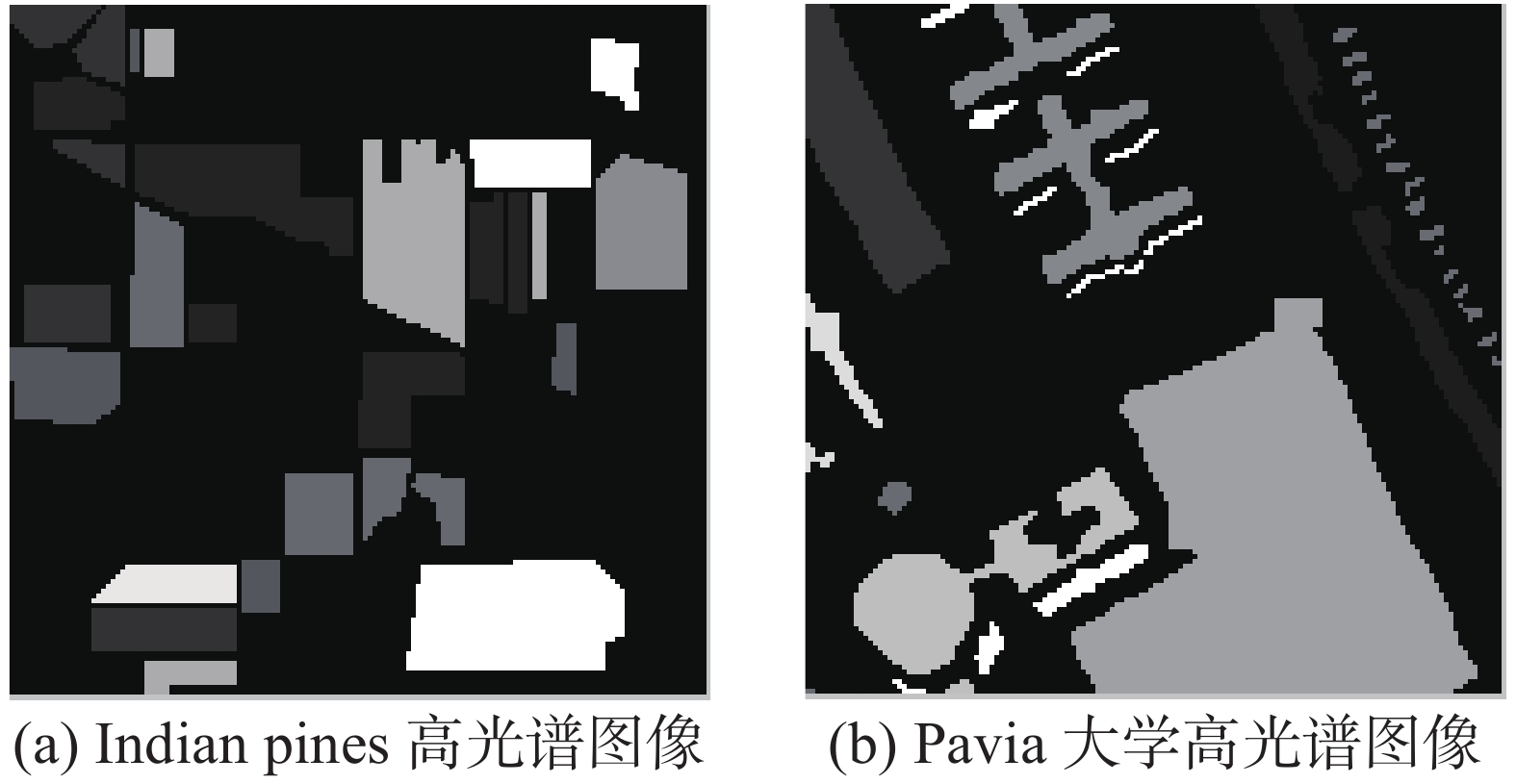Download: 图 1 监督信息

Indian pines数据集中地物的分布比较规则，每类地物的分布整体性好；而Pavia大学数据集中的同类地物分布较为分散，涉及区域较大。

3.2 实验仿真

N是样本总数，mii是第i类正确分类的样本数，Ai为第i类的分类精度。

 ${O_A} = \frac{1}{N}\sum\limits_{i = 1}^n {{m_{ii}}}$

i类分类精度为：

 $A_{i}=\frac{m_{i i}}{N_{i}}$

 ${A_A} = \frac{1}{N}\sum\limits_{i = 1}^n {{A_i}}$

Kappa系数：

 $K = \frac{{N \times \displaystyle \sum\limits_{i = 1}^n {{m_{ii}}} - \displaystyle \sum\limits_{i = 1}^n {{N_i}} \times {m_{ii}}}}{{{N^2} - \displaystyle \sum\limits_{i = 1}^n {{N_i}} \times {m_{ii}}}}$

3.3 仿真结果及其分析表 1 Indian pines高光谱图像分类精度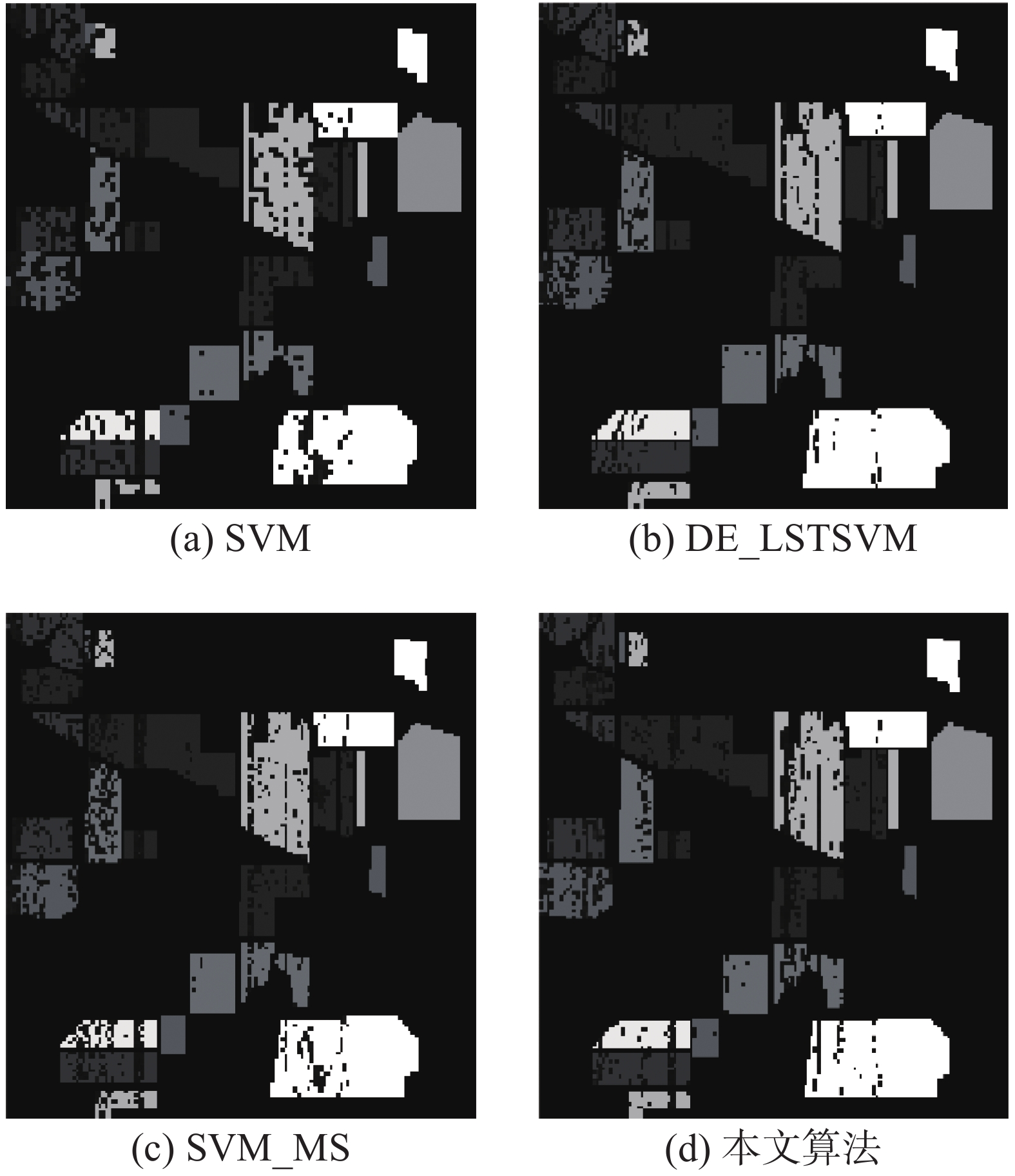Download: 图 2 Indian pines数据集高光谱图像4种算法的分类结果表 2 Pavia大学高光谱图像分类精度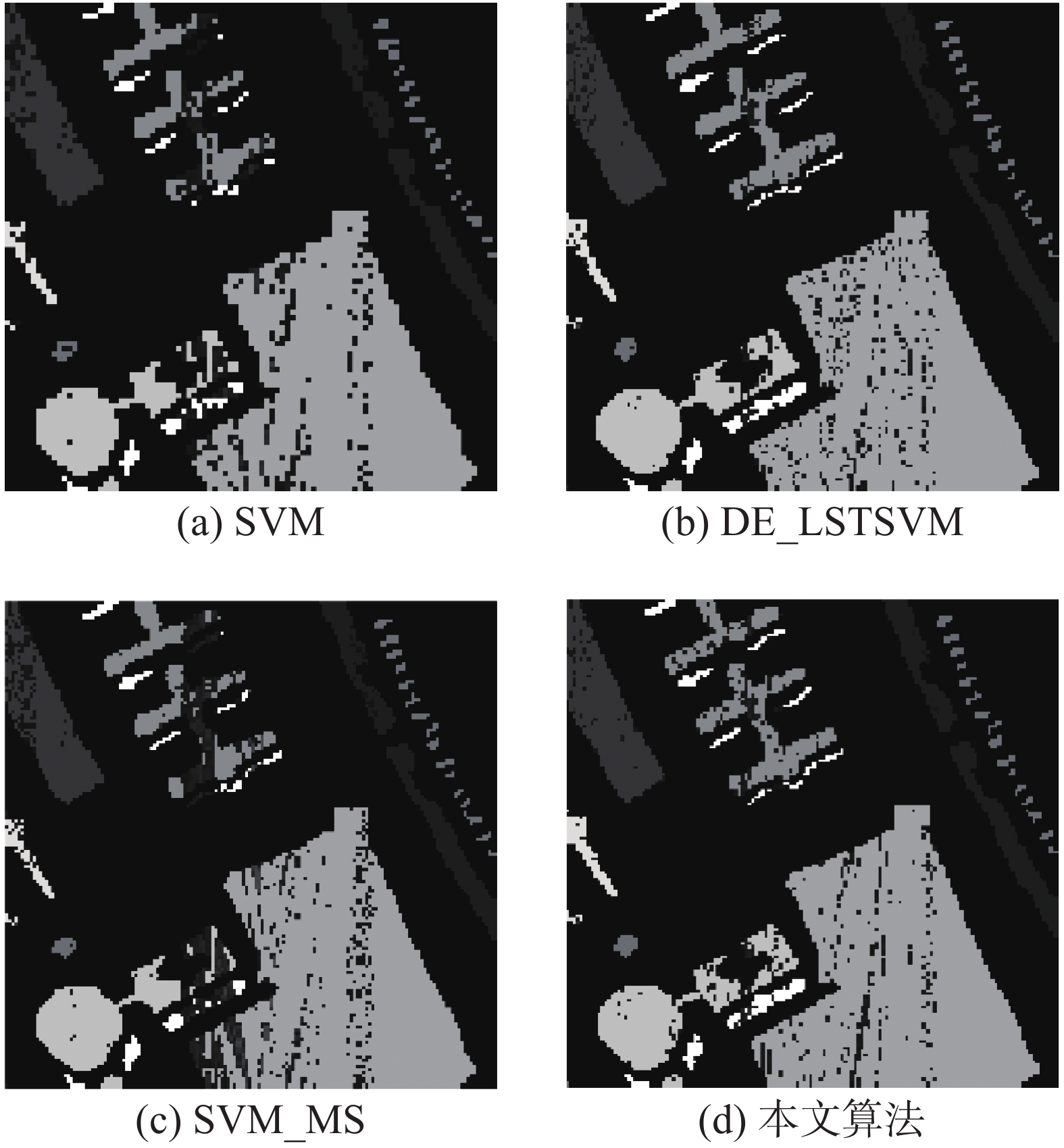Download: 图 3 Pavia大学数据集高光谱图像4种算法的分类结果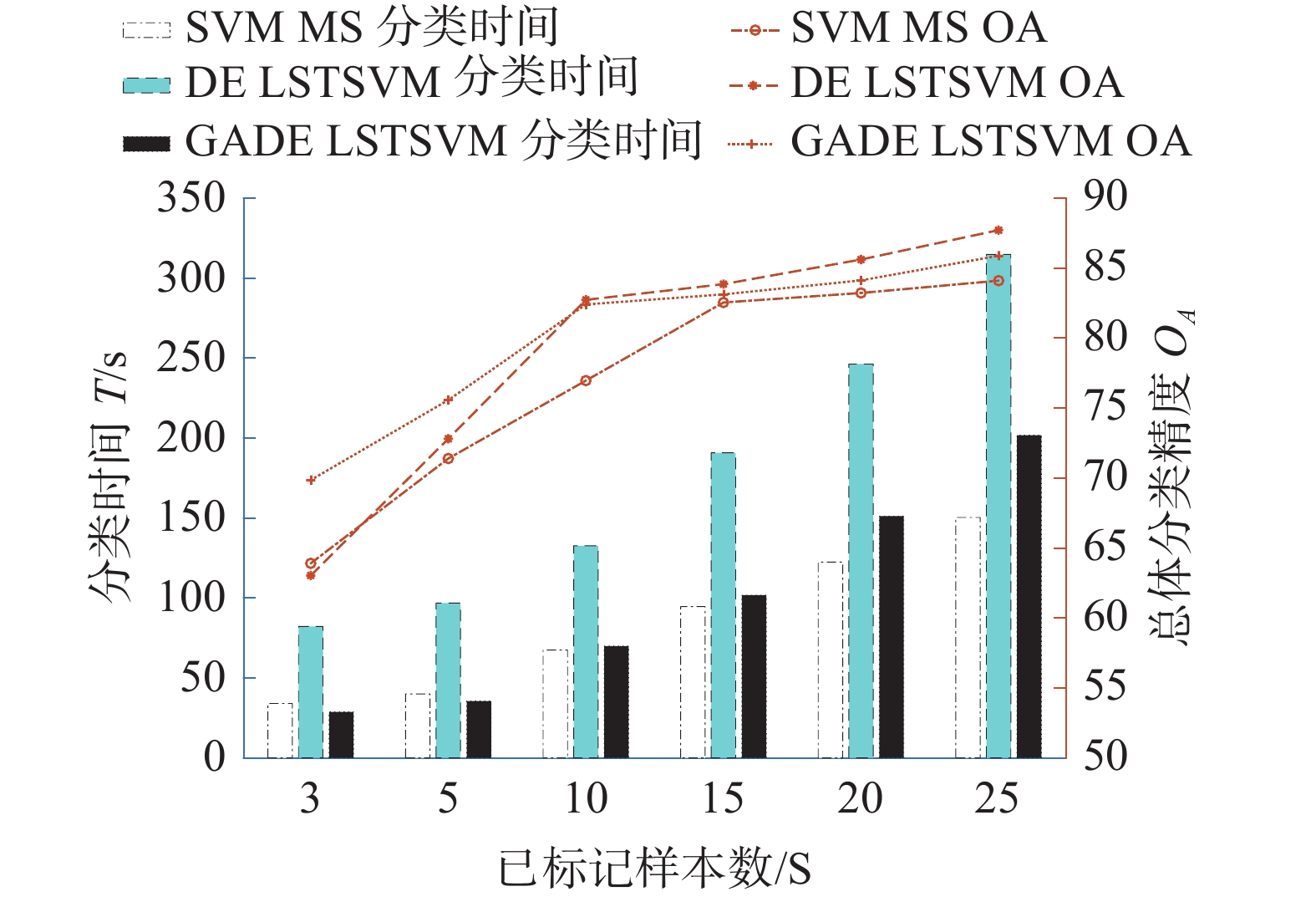Download: 图 4 带标签样本数与OA的关系曲线（Indian pines数据集高光谱图像）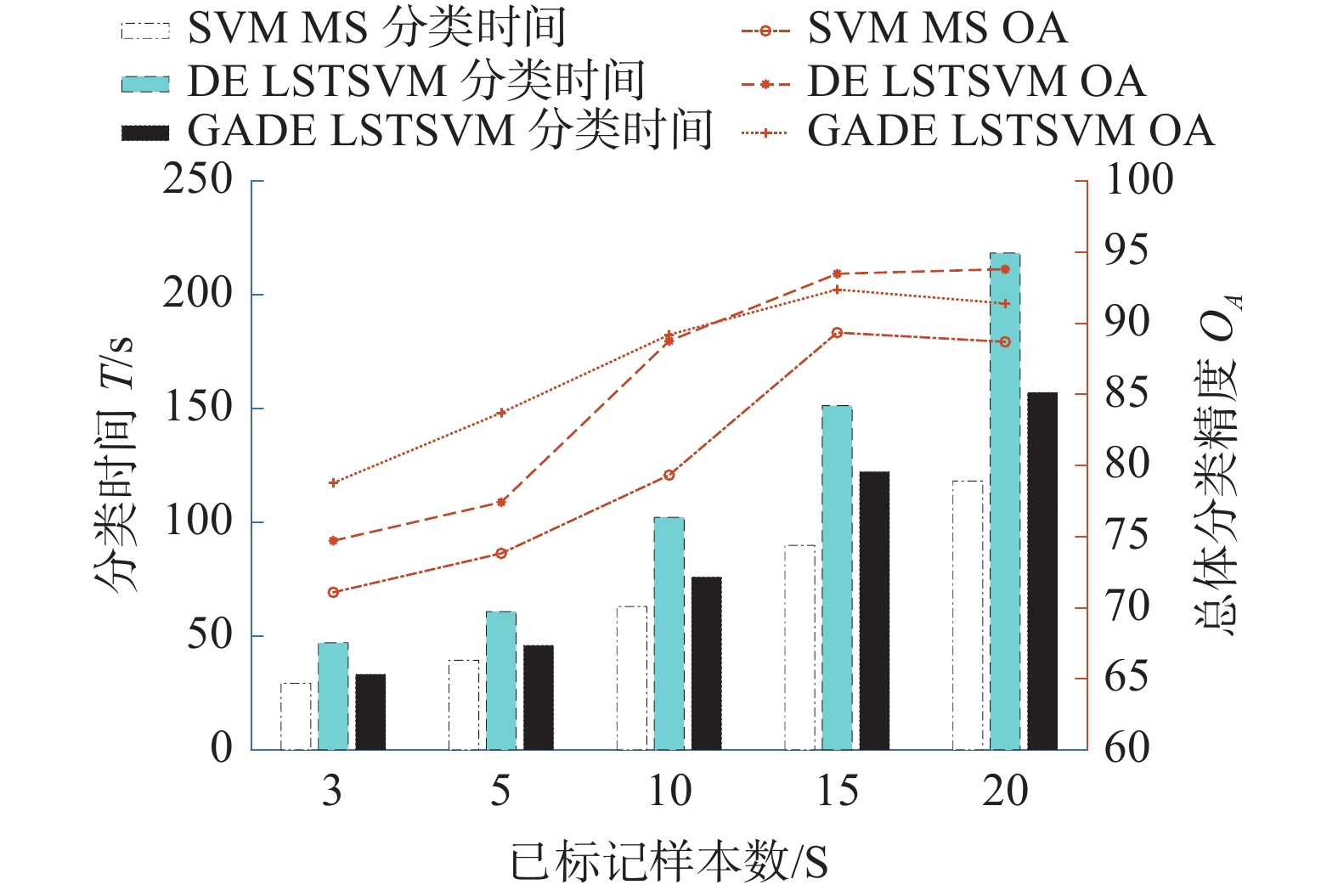Download: 图 5 带标签样本数与OA的关系曲线（Pavia大学数据集高光谱图像）
4 结论

1)本文算法在分类之前进行波段选择，有效地减少有标签样本中的冗余信息对学习过程造成的计算量。

2)在带标记样本少的情况下，本文算法能够快速地选取信息量丰富的无标签样本扩充入训练样本集中，有效地提升了分类器的性能。

3)本文算法在已标记样本数量较少时优势十分明显。

  童庆禧, 张兵, 郑兰芬. 高光谱遥感[M]. 北京: 高等教育出版社, 2006. (0)  朱艳, 刘晓莉, 杨哲海. 高光谱数据的降维及Tabu搜索算法的应用[J]. 测绘科学技术学报, 2007, 24(1): 22-25, 29. DOI:10.3969/j.issn.1673-6338.2007.01.007 (0)  ZHONG Yanfei, MA Ailong, ONG Y S, et al. Computational intelligence in optical remote sensing image processing[J]. Applied soft computing, 2018, 64: 75-93. DOI:10.1016/j.asoc.2017.11.045 (0)  CHENG Gong, HAN Junwei. A survey on object detection in optical remote sensing images[J]. ISPRS journal of photogrammetry and remote sensing, 2016, 117: 11-28. DOI:10.1016/j.isprsjprs.2016.03.014 (0)  杜培军, 夏俊士, 薛朝辉, 等. 高光谱遥感影像分类研究进展[J]. 遥感学报, 2016, 20(2): 236-256. (0)  WANG Jianyu, XUE Yongqi, RONG Shu, et al. Airborne hyperspectral and infrared remote sensing technology and application[C]//Proceedings of 2006 Joint 31st International Conference on Infrared Millimeter Waves and 14th International Conference on Teraherz Electronics. Shanghai, China, 2006. (0)  HUNG C C, KULKARNI S, KUO B C. A new weighted fuzzy c-means clustering algorithm for remotely sensed image classification[J]. IEEE journal of selected topics in signal processing, 2011, 5(3): 543-553. DOI:10.1109/JSTSP.2010.2096797 (0)  VILLA A, BENEDIKTSSON J A, CHANUSSOT J, et al. Hyperspectral image classification with independent component discriminant analysis[J]. IEEE transactions on geoscience and remote sensing, 2011, 49(12): 4865-4876. DOI:10.1109/TGRS.2011.2153861 (0)  周千琪. 基于稀疏表示和半监督主动学习的小样本高光谱数据分类问题研究[D]. 银川: 北方民族大学, 2016. (0)  高飞, 朱福利. 基于样本类别确定度的半监督分类[J]. 北京航空航天大学学报, 2018, 44(9): 1941-1951. (0)  CORTES C, VAPNIK V. Support vector networks[J]. Machine learning, 1995, 20(3): 273-297. (0)  KUMAR M A, GOPAL M. Least squares twin support vector machines for pattern classification[J]. Expert systems with applications, 2009, 36(4): 7535-7543. DOI:10.1016/j.eswa.2008.09.066 (0)  陈国良, 王煦法, 庄镇泉, 等. 遗传算法及其应用[M]. 北京: 人民邮电出版社, 1996. (0)  张明阳. 基于进化优化学习的高光谱特征选择与提取[D]. 西安: 西安电子科技大学, 2018. (0)  STORN R, PRICE K. Differential evolution—a simple and efficient heuristic for global optimization over continuous spaces[J]. Journal of global optimization, 1997, 11(4): 341-359. DOI:10.1023/A:1008202821328 (0)  刘明广. 差异演化算法及其改进[J]. 系统工程, 2005, 23(2): 108-111. DOI:10.3969/j.issn.1009-6744.2005.02.018 (0)